MINLOC and MAXLOC

## MINLOC and MAXLOC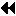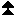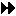Up: Global Reduction Operations Next: User-Defined Operations Previous: Predefined reduce operations

The operator MPI_MINLOC is used to compute a global minimum and also an index attached to the minimum value. MPI_MAXLOC similarly computes a global maximum and index. One application of these is to compute a global minimum (maximum) and the rank of the process containing this value.

The operation that defines MPI_MAXLOC is: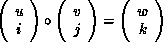where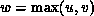and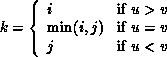MPI_MINLOC is defined similarly:where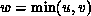and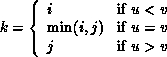Both operations are associative and commutative. Note that if MPI_MAXLOC is applied to reduce a sequence of pairs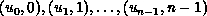, then the value returned is (u , r), where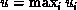and r is the index of the first global maximum in the sequence. Thus, if each process supplies a value and its rank within the group, then a reduce operation with op = MPI_MAXLOC will return the maximum value and the rank of the first process with that value. Similarly, MPI_MINLOC can be used to return a minimum and its index. More generally, MPI_MINLOC computes a lexicographic minimum, where elements are ordered according to the first component of each pair, and ties are resolved according to the second component.

The reduce operation is defined to operate on arguments that consist of a pair: value and index. For both Fortran and C, types are provided to describe the pair. The potentially mixed-type nature of such arguments is a problem in Fortran. The problem is circumvented, for Fortran, by having the MPI-provided type consist of a pair of the same type as value, and coercing the index to this type also. In C, the MPI-provided pair type has distinct types and the index is an int.

In order to use MPI_MINLOC and MPI_MAXLOC in a reduce operation, one must provide a datatype argument that represents a pair (value and index). MPI provides seven such predefined datatypes. The operations MPI_MAXLOC and MPI_MINLOC can be used with each of the following datatypes.
[ Fortran:]
[ Name] Description
[ MPI_2REAL]pair of REALs
[ MPI_2DOUBLE_PRECISION]pair of DOUBLE PRECISION variables
[ MPI_2INTEGER]pair of INTEGERs

[ C:]
[ Name] Description
[ MPI_FLOAT_INT] float and int
[ MPI_DOUBLE_INT] double and int
[ MPI_LONG_INT] long and int
[ MPI_2INT]pair of int
[ MPI_SHORT_INT] short and int
[ MPI_LONG_DOUBLE_INT] long double and int

The datatype MPI_2REAL is as if defined by the following (see Section Derived datatypes ).

```MPI_TYPE_CONTIGUOUS(2, MPI_REAL, MPI_2REAL)
```
Similar statements apply for MPI_2INTEGER, MPI_2DOUBLE_PRECISION, and MPI_2INT.

The datatype MPI_FLOAT_INT is as if defined by the following sequence of instructions.

```type = MPI_FLOAT
type = MPI_INT
disp = 0
disp = sizeof(float)
block = 1
block = 1
MPI_TYPE_STRUCT(2, block, disp, type, MPI_FLOAT_INT)
```

Similar statements apply for MPI_LONG_INT and MPI_DOUBLE_INT.

```

Each process has an array of 30  doubles, in C.  For each
of the 30 locations, compute the value and rank of the process containing
the largest value.

...
/* each process has an array of 30 double: ain
*/
double ain, aout;
int  ind;
struct {
double val;
int   rank;
} in, out;
int i, myrank, root;

MPI_Comm_rank(MPI_COMM_WORLD, &myrank);
for (i=0; i<30; ++i) {
in[i].val = ain[i];
in[i].rank = myrank;
}
MPI_Reduce( in, out, 30, MPI_DOUBLE_INT, MPI_MAXLOC, root, comm );
/* At this point, the answer resides on process root
*/
if (myrank == root) {
*/
for (i=0; i<30; ++i) {
aout[i] = out[i].val;
ind[i] = out[i].rank;
}
}

```

```

Same example, in Fortran.

...
! each process has an array of 30 double: ain(30)

DOUBLE PRECISION ain(30), aout(30)
INTEGER ind(30);
DOUBLE PRECISION in(2,30), out(2,30)
INTEGER i, myrank, root, ierr;

MPI_COMM_RANK(MPI_COMM_WORLD, myrank);
DO I=1, 30
in(1,i) = ain(i)
in(2,i) = myrank    ! myrank is coerced to a double
END DO

MPI_REDUCE( in, out, 30, MPI_2DOUBLE_PRECISION, MPI_MAXLOC, root,
comm, ierr );
! At this point, the answer resides on process root

IF (myrank .EQ. root) THEN
DO I= 1, 30
aout(i) = out(1,i)
ind(i) = out(2,i)  ! rank is coerced back to an integer
END DO
END IF

```

```

Each process has a non-empty array of values.
Find the minimum global value, the rank of the process that holds it
and its index on this process.

#define  LEN   1000

float val[LEN];        /* local array of values */
int count;             /* local number of values */
int myrank, minrank, minindex;
float minval;

struct {
float value;
int   index;
} in, out;

/* local minloc */
in.value = val;
in.index = 0;
for (i=1; i < count; i++)
if (in.value > val[i]) {
in.value = val[i];
in.index = i;
}

/* global minloc */
MPI_Comm_rank(MPI_COMM_WORLD, &myrank);
in.index = myrank*LEN + in.index;
MPI_Reduce( in, out, 1, MPI_FLOAT_INT, MPI_MINLOC, root, comm );
/* At this point, the answer resides on process root
*/
if (myrank == root) {
*/
minval = out.value;
minrank = out.index / LEN;
minindex = out.index % LEN;
}

```

[] Rationale.

The definition of MPI_MINLOC and MPI_MAXLOC given here has the advantage that it does not require any special-case handling of these two operations: they are handled like any other reduce operation. A programmer can provide his or her own definition of MPI_MAXLOC and MPI_MINLOC, if so desired. The disadvantage is that values and indices have to be first interleaved, and that indices and values have to be coerced to the same type, in Fortran. ( End of rationale.)Up: Global Reduction Operations Next: User-Defined Operations Previous: Predefined reduce operations